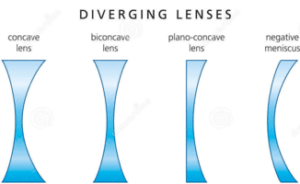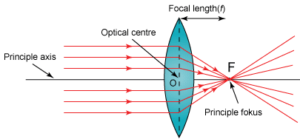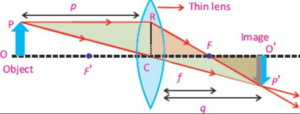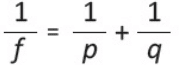Optics

# Types of Lenses

Lenses are the pieces of transparent material of which one or both sides are spherical. It is any transparent material having two surfaces, of which at least one is curved. Lenses refract light in such a way that an image of the object is formed. Each surface of a lens is a part of a sphere. Common types of lenses, the convex lenses, concave lenses and, contact lenses.

You know that when light passes from one transparent medium to another, it changes its direction. This is called refraction. Who is not aware of the use of lenses in the spectacles? Many people wear glasses so that they can see clearly. Lenses are commonly used in cameras, telescopes, microscopes and film projectors. There are also two lenses in our eyes through which we see around us.

Lenses of many different types are used in optical devices such as cameras, eyeglasses, microscopes, telescopes, and projectors. They also enable millions of people to see clearly and read comfortably.

## Types of Lenses physics

Convex lens and concave lens are two basic types of lenses:

### Convex lenses

“The lens which causes incident parallel rays to diverge at a point is known as a convex or converging lens. This lens is thick at the center but thin at the edges.”

### Concave lenses“Another type of lens causes the parallel rays of light to diverge from a point. This is called a concave or diverging lens. This lens is thin at the center and thick at the edges.”

## Difference between the convex and concave lens in tabular form

 Convex lens Concave lens It is converging lens. It is diverging lens. It forms real image. It forms virtual image. Its principal focus is real. Its principle focus is virtual. It is thick in middle. It is thick at corners. It is thin at corners. It is thin at middle.

## Lens Terminology### Principal Axis:

Each of the two surfaces of a spherical lens is a section of a sphere. The line passing through the two centers of curvatures of the lens is called principal axis.

### Optical Center(C):

A point of the principal axis at the center of the lens is called the optical center.

### Principle Focus (F):

The light rays traveling parallel to the principal axis of a convex lens after refraction meet at a point on the principal axis, called the principal focus of focal point F. Hence, a convex lens is also called a converging lens. A concave lens is called principal focus F . Hence concave lens is also called a diverging lens.

### Focal length (f):

This is the distance between the optical center and the principal focus.

## Power of a lens

The Power of a lens is defined as the reciprocal of its focal length in meters. Thus:

Power of a lens = p = 1/focal length in meters

The SI unit of power of a lens is “Dioptre”, denoted by a symbol D. If f is expressed in meters 1D = 1m-1. Thus,1 Dioptre is the power of a lens whose focal length is 1 meter. Because the focal length of a convex lens is positive, therefore, its power is also positive. Whereas the power of a concave lens is negative, for it has a negative focal length.

## Image location by lens equationLet an object OP is placed in front of a convex lens at a distance of p. A ray PR parallel to the principal axis after refraction passes through focus F. Another ray PC meets the first ray at point P after passing through the optical center C. If this process is repeated for the other points of the object, a real and inverted image OP‘ is formed at a distance q from the lens.

What is the size of the image formed in a lens for a particular distance of the object from the lens? What is the nature of the image,i.e., whether the image is real or imaginary, erect or inverted? A lens formula is a tool that we use to answer all such questions. We define the lens formula as:

The relation between the object and image distance from the lens in terms of the focal length of the lens is called the lens formula.## Sign conventions for lenses:## Magnification

“The ratio of the size of the image to the size of the object is called magnification.”When an object is placed in front of a convex lens at a point beyond its focus, a real and inverted image of the object is formed.

As the object is brought from a far-off point to the focus, the magnification goes on to increase. The apparent size of an object depends on the angle subtended by it at the eye. Thus, the closer the object is to the eye, the greater is the angle subtended and the larger appears the size of the object. The maximum size of an object as seen by naked eye is obtained when the object is placed at the least distance of distinct vision. For lesser distances, the image formed looks blurred and the details of the object are not visible.

## Magnifying power

“The magnifying power or angular magnification is defined as the ratio of the angles subtended by the image as seen through the optical device to that subtended by the object at the unaided eye.”

The optical resolution of a microscope or a telescope tells us how close together the two point sources of light can be so that they are still seen as two separate sources.If two point sources are too close, they will appear as one because the optical instrument makes a point source look like a small disc or spot of light with circular diffraction fringes.

Although the magnification can be made as large as one desire by choosing appropriate focal lengths, the magnification alone is of no use unless we can see the details of the object distinctly.

## Resolving power

“The resolving power of an instrument is its ability to reveal the minor details of the object under examination.”

Resolving power is expressed as the reciprocal of minimum angle which two point sources subtend at the instrument so that their images are seen as two distinct spots of light rather than one. Raleigh showed that for light of wavelength λ through a lens of diameter D, the resolving power is given by:

R =1/αmin =D/1.22λ

Where αmin =1.22 λ/D

The smaller the value of αmin , greater is the resolving power because two distant objects which are close together can then be seen separated through the instrument. In the case of a grating spectrometer , the resolving power R of the grating is defined as:

R = λ/λ2– λ1 =λ/Δλ

Where λ ≈ λ1 ≈ λand Δλ = λ2– λ1 . Thus, we see that a grating with high resolving power can distinguish the small differences in wavelength. If N is the number of rulings on the grating, it can be shown that the resolving in the mth order diffraction equals the product N × m,i.e.

R =N ×m

## Uses of lenses in daily life

Applications of lenses in some optical devices such as cameras, slide projectors, and photograph enlargers.

### Application of lenses in camera

A camera is a kind of box in which a convex lens is mounted on the front side. The lens forms the real and inverted image of an object on the sensitive film placed behind it. A system is provided in the camera to move the lens back and forth so that a clear image is obtained on the film. There is a shutter close to the lens that remains shut normally. When the button is pressed, the shutter opens for a small interval and then is closed again. Light enters the camera during this interval and the image of the object in front of the camera is formed on the film.The amount of light entering into the camera depends upon the size of the aperture. The aperture is an opening in the diaphragm. This can be made smaller or larger as needed. The picture is obtained by developing the image on the film.

### Lens Camera and human eye

The human eye also works like a camera. The eye is almost a sphere of a diameter of about 2.5 centimeters. Its outer boundary called the sclera is thick and hard. At the front of the eye, there is a transparent hard skin known as a cornea. Behind the cornea, there is an iris and after that, there is a convex lens. The inner layer of the black wall of the eye is called the retina.The retina of the eye and the film of the camera serves the same purpose. Like a camera, the eye lens forms a real and inverted image of the object on the retina. The optic nerve carries it in the form of signals to the brain. Although the image formed on the retina is inverted, but our brain interprets this correctly i.e the right way up.

#### Accommodation

“The variation of the focal length of the eye lens to form a sharp image on the retina is called accommodation.”The camera focuses the image of an object at a given distance from it by moving the lens towards or away from the film. The eye has different adjusting mechanisms for focusing the image of an object onto the retina. Its ciliary muscles control the curvature and thus the focal length of the lens, and allow objects at various distances to be seen.

If an object is far away from the eye, the deviation of light through the lens must be less.To do this, the ciliary muscles relax and decreases the curvature of the lens, thereby, increasing the focal length. The rays are thus focused onto the retina producing a sharp image of the distant object.

If an object is close to the eye, the ciliary muscles increase the curvature of the lens, thereby, shortening the focal length. The divergent rays from the nearer object are thus bent more so as to come to a focus on the retina. It is large in young people while it goes on decreasing with age. Defects in accommodation may be corrected by using different types of lenses in eyeglasses. In the following sections, we will describe the defects of vision and their remedies.

### Slide projectorFigure shows how a slide or movie projector works. The light source is placed at the center of curvature of a converging or concave mirror. The concave mirror is used to reflect light back in fairly parallel rays. The condenser is made up of 2 converging lenses that refract the light so all parts of the slide are illuminated with parallel rays.

The projection or converging lens provides a real, large and inverted image. It must be real to be projected on a screen. The slide (object) must be placed between F and 2F of the projection lens so as to produce a real, large, and inverted image. Because the image is inverted, the slide must be placed down and laterally inverted so we can see the image properly.

### Photograph enlarger

In the case of a photograph enlarger object is placed at a distance of more than F but less than 2 F. In this way, we get a real, inverted and enlarged image. The working principle of a photograph enlarger is basically the same as that of a slide projector. It uses a convex lens to produce a real, magnified, and inverted image of the film on photographic paper.

### Related Articles

1.Clever banda says: